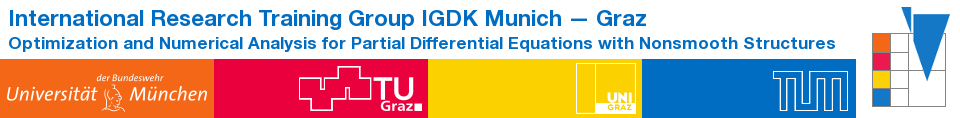IGDK1754QualificationProgramCompactCoursesCC_Tropp

Concentration inequalities and matrix computations

Prof. Joel A. Tropp, Caltech

Course content

This live online course gives an introduction to the theory of concentration inequalities with some basic applications to matrix computations. The course assumes only a basic level of experience with probability, linear algebra, and matrix computations. It does not assume exposure to high-dimensional probability or randomized matrix computations. The course will consist of four 90-minute lectures:

Monday, 3 May, 18:30 - 20:00

Scalar concentration: Independent sum model. Markov's inequality, cumulant generating functions, Laplace transform method, Bernstein inequality. Application to trace estimation.

Tuesday, 4 May, 18:30 - 20:00

Matrix concentration: Independent sum model. Matrix cumulant generating functions, matrix Laplace transform method, matrix Bernstein inequality. Application to approximate matrix multiplication.

Wednesday, 5 May, 18:30 - 20:00

Subspace embeddings: Random projections. Oblivious subspace embeddings. Examples: Sampling, sign matrices, Gaussian matrices. Application to linear regression.

Thursday, 6 May, 18:30 - 20:00

Randomized SVD: The truncated SVD. Randomized SVD algorithm. Linear algebraic error bound. Probabilistic error bound. Randomized subspace iteration.

Registration

Registration for this course is required. Registration is closed. All participants receive a confirmation certificate for 6 credit hours.
Please note: You must login to the course using your full name for the certificate to be issued.

This course is based on the following references:

N. Halko, P.-G. Martinsson, and J. A. Tropp: Finding structure with randomness: Probabilistic algorithms for constructing approximate matrix decompositions, SIAM Review, 53 (2011), pp. 217288.

J. A. Tropp: An Introduction to Matrix Concentration Inequalities, Found. Trends Mach. Learning, 8 (2015), pp. 1230

J. A. Tropp: Matrix concentration and computational linear algebra, Caltech CMS Lecture Notes 2019-01

P.-G. Martinsson and J. A. Tropp: Randomized numerical linear algebra: Foundations and algorithms, Acta Numerica, 29 (2020), pp. 403572

J. A. Tropp: Randomized algorithms for matrix computations, Caltech CMS Lecture Notes 2020-01.

Biography:

Joel A. Tropp is Steele Family Professor of Applied and Computational Mathematics at Caltech. His research centers on data science, applied mathematics, numerical algorithms, and random matrix theory. He attained the Ph.D. degree in Computational Applied Mathematics at the University of Texas at Austin in 2004, and he joined Caltech in 2007. Prof. Tropp won the PECASE in 2008, and he was recognized as a Highly Cited Researcher in Computer Science each year from 20142018. He is co-founder and Section Editor of the SIAM Journal on Mathematics of Data Science (SIMODS), and he was co-chair of the inaugural 2020 SIAM Conference on the Mathematics of Data Science. Prof. Tropp was elected SIAM Fellow in 2019 and IEEE Fellow in 2020.

-- DianeClaytonWinter - 27 Apr 2021